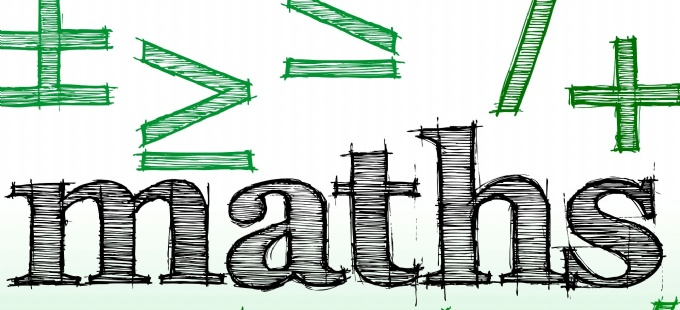# MathsThis week we have been looking at methods for multiplication.

We started the week by looking at using mental methods to solve problems such as 24 x 6 and 38 x 7. We looked at 4 different methods to begin with but as a class we all decided that there was one method that we all found easier.

We started by looking at the 2-digit number in our calculation 38 x 7.

In this case it's 38. We then partitioned it to get 30 and 8.

We then worked out 30 x 7 and 8 x 7; either in our heads or written in our books.

38 x 7 =

30 x 7 = 210 (if we found this tricky we started with 3 x 7 and then added the 0 back on).

8 x 7 = 56

210 + 56 = 266 (we used column addition in our books).

We then moved on to using the short method for multiplying 3-digits by single digit numbers (this is the method you may remember from your school days!). This website link below is a great way to practise if needed.

Multiply a 3-digit number by a 1-digit number - Year 5 - P6 - Maths - Home Learning with BBC Bitesize - BBC Bitesize

Why don't you try a few together at home and see who gets the most right answers!

Ready: 34 x 6, 64 x 5, 79 x 3

Steady: 321 x 5, 347 x 3, 265 x 9

Go: 753 x 4, 435 x 6, 623 x 6